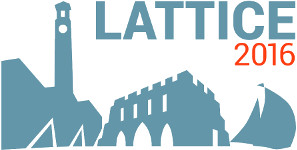#The 34th International Symposium on Lattice Field Theory (Lattice 2016)

Jul 24 – 30, 2016
Highfield Campus, University of Southampton
Europe/London timezone

## long distance part of $\epsilon_K$ from lattice QCD

Jul 28, 2016, 5:30 PM
20m
Building 67 Room 1003 (Highfield Campus, University of Southampton)

### Building 67 Room 1003

#### Highfield Campus, University of Southampton

Highfield Campus, Southampton SO17 1BJ, UK
Talk Weak Decays and Matrix Elements

### Speaker

Mr Ziyuan Bai (Columbia)

### Description

We demonstrate the lattice QCD calculation of the long distance contribution to $\epsilon_K$. Due to the singular, short-distance structure of $epsilon_K$, we must perform a short-distance subtraction and introduce a corresponding subtraction term determined from perturbation theory, which we calculate at Next Leading Order (NLO). We perform the calculation on a $24^3 \times 64$ lattice with a pion mass of 329 MeV. This work is a complete calculation, which includes all connected and disconnected diagrams.

### Primary author

Mr Ziyuan Bai (Columbia)

 Slides